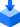Surface Mount Type Aluminum Electrolytic CapacitorsDownload all(4.56MB)

Frequency characteristic data, equivalent circuit models, and S-parameter data can be downloaded for each individual item number.

View All

* 1) The H size of vibration-proof products is the following size + 0.3mm

Series Standard Part Number Vibration-proof Part Number Rated voltage
V
Capacitance
uF
ESRmohm
(100kHz)
Rated ripple current
mAr.m.s
(100kHz)
Diameter
ømm
*1)
Body height
Hmm
Simulation Data
（Frequency characteristic data/equivalent circuit models/S-parameter data）